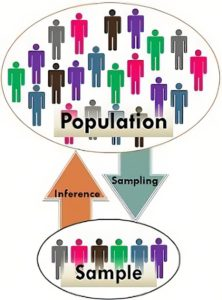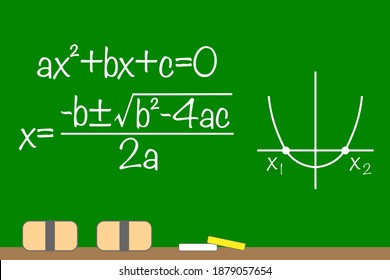# MS Math April 25-29, 20226th Grade Math – Mrs. Evans

This week we will finish Chapter 10.   We will be able to calculate the area and perimeter of most simple forms – squares, rectangles, parallelograms, triangles, trapezoids and regular polygons.  We can use these simple shapes to model composite shapes in order to find the area of these figures.  By knowing how to find the area of simple shapes we can use our critical thinking to apply it to many shapes we see.  Here is a video showing how to apply simple shape formula to find the area of composite shapes to find the area and the perimeter:

Math Grade Math – Mrs. Evans and Mrs. VonFeldt

This week, the 7th graders will be finishing Module 10. In Module 10, we have understood that when we want to study a population of something, we can study a random sample of that population, evaluate the data, and make inferences (predictions or educated guesses).  This is very useful in the real world.  This is an overall picture of how the process works:Algebra – Mrs. VonFeldt

This week we will learn the last two strategies for solving quadratic equations: Completing the Square and the Quadratic Formula. The Quadratic Formula can be used for any quadratic equation, however, it is particularly useful when the “a” “b” and “c” terms are not integers. It is so useful in fact, that it is worth spending the time to memorize it. (see the song below). We will spend time this week reviewing all the strategies we have learned and determining which one is the best for a particular equation. There will be a test on Chapter 9 next week.

QUADRATIC EQUATION:© 2023 Assumption Catholic School | 2116 Cornwall Ave, Bellingham, WA 98225
Phone: 360.733.6133 | Fax: 360.647.4372
Email: theoffice@school.assumption.org
Dashboard | Web design and development by Olywebdev.com